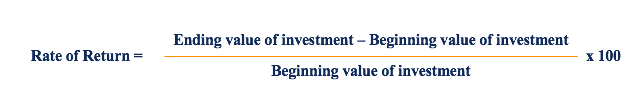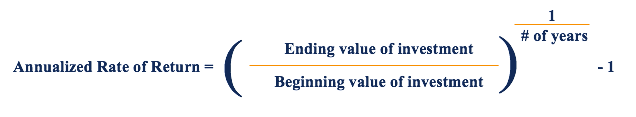# Rate of Return

The gain or loss of an investment over a certain period

## What is a Rate of Return?

A Rate of Return (ROR) is the gain or loss of an investment over a certain period of time. In other words, the rate of return is the gain (or loss) compared to the cost of an initial investment, typically expressed in the form of a percentage. When the ROR is positive, it is considered a gain and when the ROR is negative, it reflects a loss on the investment.

### Video Explanation of Rate of Return

Watch this short video to quickly understand the main concepts covered in this guide, including the definition of rate of return, the formula for calculate ROR and annualized ROR, and example calculations.

### Formula for Rate of Return

The standard formula for calculating ROR is as follows:Keep in mind that any gains made during the holding period of the investment should be included in the formula. For example, if a share costs \$10 and its current price is \$15 with a dividend of \$1 paid during the period, the dividend should be included in the ROR formula. It would be calculated as follows:

### Example Rate of Return Calculation

Adam is a retail investor and decides to purchase 10 shares of Company A at a per-unit price of \$20. Adam holds onto shares of Company A for 2 years. In that time frame, Company A  paid yearly dividends of \$1 per share. After holding them for 2 years, Adam decides to sell all 10 shares of Company A at an ex-dividend price of \$25. Adam would like to determine the rate of return during the two years he owned the shares.

To determine the rate of return, first calculate the amount of dividends he received over the two-year period:

##### 10 shares x (\$1 annual dividend x 2) = \$20 in dividends from 10 shares

Next, calculate how much he sold the shares for:

##### 10 shares x \$25 = \$250 (Gain from selling 10 shares)

Lastly, determine how much it cost Adam to purchase 10 shares of Company A:

##### 10 shares x \$20 = \$200 (Cost of purchasing 10 shares)

Plug all the numbers into the rate of return formula:

##### = ((\$250 + \$20 – \$200) / \$200) x 100 = 35%

Therefore, Adam realized a 35% return on his shares over the two-year period.

### Annualized Rate of Return

Note that the regular rate of return describes the gain or loss, expressed in a percentage, of an investment over an arbitrary time period. The annualized ROR, also known as the Compound Annual Growth Rate (CAGR), is the return of an investment over each year.

### Formula for Annualized ROR

The formula for annualized ROR is as follows:Similar to the simple rate of return, any gains made during the holding period of this investment should be included in the formula.

### Example of Annualized Rate of Return

Let us revisit the example above and determine the annualized ROR. Recall that Adam purchased 10 shares at a per-unit price of \$20, received \$1 in dividends per share each year, and sold the shares at a price of \$25 after 2 years. The annualized ROR would be as follows:

### Alternative Measures of Return

Return can mean different things to different people, and it’s important to know the context of the situation to understand what they mean.  In addition to the above methods for measuring returns, there several other types of formulas.

Common alternative types of returns include:

• Internal Rate of Return (IRR)
• Return on Equity (ROE)
• Return on Assets (ROA)
• Return on Investment (ROI)
• Return on Invested Capital (ROIC)

### More Resources

CFI is the official provider of the Financial Modeling & Valuation Analyst (FMVA) certification, designed to teach valuation modeling skills to financial analysts.  To continue advancing your career, these additional resources will be useful:

• Investing: A Beginner’s Guide
• Unlevered Beta (Asset Beta)
• Basis Points (bps)
• Technical Analysis: A Beginner’s Guide

### Financial Analyst Training

Get world-class financial training with CFI’s online certified financial analyst training program!

Gain the confidence you need to move up the ladder in a high powered corporate finance career path.

Learn financial modeling and valuation in Excel the easy way, with step-by-step training.